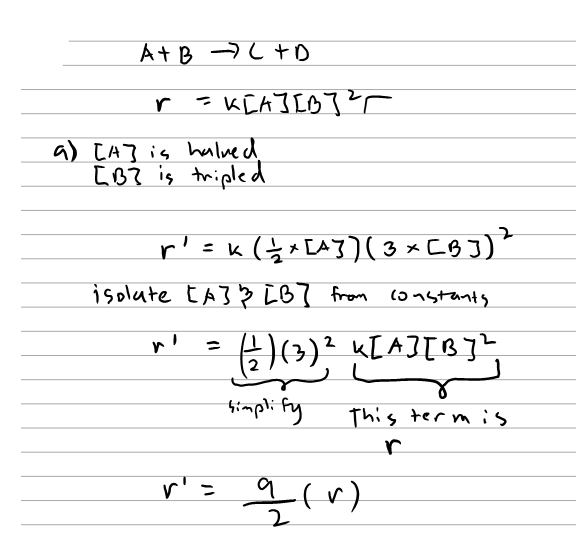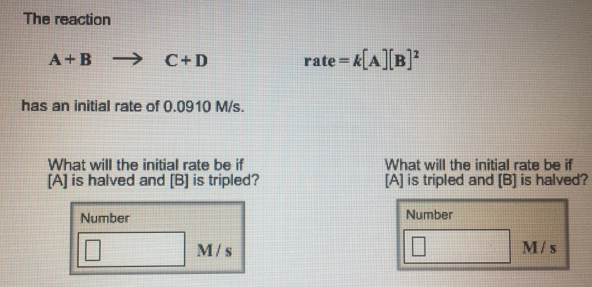# Problem: The reaction A + B → C + D                                rate = k[A][B]2 has an initial rate of 0.0910 M/s. What will the initial rate be if [A] is halved and [B] is tripled? What will the initial rate be if [A] is tripled and [B] is halved?

###### FREE Expert Solution87% (103 ratings)###### Problem Details

The reaction

A + B → C + D                                rate = k[A][B]2

has an initial rate of 0.0910 M/s.

What will the initial rate be if [A] is halved and [B] is tripled?

What will the initial rate be if [A] is tripled and [B] is halved?